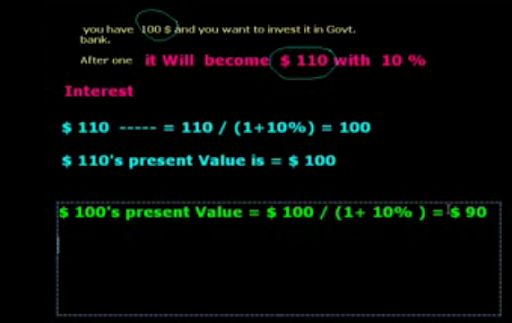Today, we will learn a very important topic and its name is present value. If you invest in any investment proposal. Suppose, if you want to invest your money in machinery. There are many alternatives in the market. But you are seeing which is the best and which gives you better return. For this, you will calculate net present value (NPV) of cash flows of both machines. You should purchase only that machine that has high NPV. So, first of all we should know what present value is. Present value is the today value of future money.

Suppose, you have to invest your 100\$ in Govt. bank. After one year, it will become \$110 with 10% interest rate. So, \$110's present Value is \$ 100. Because, after one year, \$100 will convert \$ 110.

We can calculate present value of any amount with any discount rate with simple formula

PV = Amount / (1+r)

The present Value for all the cash inflows for a number of years is thus found as follows.

```{ Note: A1, A2, A3……… An are future net cash flows

r = rate of interest

2, 3 ……… n are number of years} ```.

## \$type=three\$a=hide\$cm=hide\$s=hide\$show=/2019/06/10-steps-to-become-better-investor.html\$l=hide

Name

false
ltr
item
Accounting Education: Present Value
Present Value
http://lh6.ggpht.com/_DJEIRrK4tl4/S50m9Y_joPI/AAAAAAAAEyc/GIFQfGdEOVA/present%20value.PNG
http://lh6.ggpht.com/_DJEIRrK4tl4/S50m9Y_joPI/AAAAAAAAEyc/GIFQfGdEOVA/s72-c/present%20value.PNG
Accounting Education
http://www.svtuition.org/2010/03/present-value.html
http://www.svtuition.org/
http://www.svtuition.org/
http://www.svtuition.org/2010/03/present-value.html
true
2410664366776677676
UTF-8